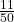## Write as a fraction or mixed numbers. 7.22

Question

Write as a fraction or mixed numbers.

7.22

in progress 0
7 months 2021-08-17T11:27:34+00:00 2 Answers 0 views 0

1. for fraction form 361/50 you can simplify from there

361/50 or 7Step-by-step explanation:

7.22 = 722/100 = 361/50 = 7 11/50

The number 7.22 can be written using the fraction 722/100 which is equal to 361/50 when reduced to lowest terms.

It is also equal to 7 11/50 when written as a mixed number.

You can use the following approximate value(s) for this number:

7.22 =~ 7 2/9 (if you admit an error of = 0.030779%)

361/50 =~ 7 1/4 (if you admit an error of 0.415513%)

7.22 =~ 7 (if you admit an error of -3.047091%)